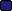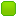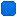###Author Topic: SCI0 - Graph fill box and Dithering  (Read 1499 times)

0 Members and 1 Guest are viewing this topic.

####gumby

• Moderator
• SCI Guru
••• Posts: 1030
• 7543.00 credits
• View Inventory
• Send Money To gumby
• Karma: +6/-0##### SCI0 - Graph fill box and Dithering
« on: November 27, 2016, 10:58:59 AM »
The Graph() kernel method doesn't support using a dithered color.  So to overcome this I wrote this procedure:

Code: [Select]
`(procedure (GraphDither y1 x1 y2 x2 clColor1 clColor2)   (var xI, xII, yI, yII, yOdd)             Graph(grFILL_BOX y1 x1 y2 x2 VISUAL clColor1)                = yOdd 0   (if (<> (% (- y2 y1) 2) 0)     = yOdd 1   )     = xI x1   = yI (- y2 2)   = xII (- (+ x1 2) 1)   = yII (- y2 1)            (if (yOdd)      = yI (- y2 1)      = xII x1     )   (while (1)             (if ((>= xII x2))                = xII (- x2 1)                = yII (- yII 1)       )             Graph(grDRAW_LINE yI xI yII xII clColor2)             (if (<= (+ xII 2) x2)     = xII (+ xII 2)       )(else   = yII (- yII 2)       )         (if (>= (- yI 2) y1)     = yI (- yI 2)       )(else       = xI (+ xI 2)               )       (if (>= xI x2)    break       )   ))`
The procedure starts by drawing a box with the first specified color, then draws parallel lines (slope = 1) which colors every other pixel in the box with the 2nd dither color.

In the Great Underground Empire (Zork port in development)

####troflip

• SCI maestro
• Moderator
• SCI Guru
••• Posts: 1525
• 12031.00 credits
• View Inventory
• Send Money To troflip
• Karma: +32/-0##### Re: SCI0 - Graph fill box and Dithering
« Reply #1 on: November 27, 2016, 11:37:16 AM »
Clever.

It's too bad Graph doesn't support dithering natively (your technique won't get undithered in ScummVM, for instance).

Here it is in Sierra syntax, which is what we should be posting snippets in (it's trivial to convert automatically in Companion):

Code: [Select]
`(procedure (GraphDither y1 x1 y2 x2 clColor1 clColor2 &tmp xI xII yI yII yOdd) (Graph grFILL_BOX y1 x1 y2 x2 VISUAL clColor1) (= yOdd 0) (if (!= (mod (- y2 y1) 2) 0) (= yOdd 1)) (= xI x1) (= yI (- y2 2)) (= xII (- (+ x1 2) 1)) (= yII (- y2 1)) (if yOdd (= yI (- y2 1)) (= xII x1)) (repeat (if (>= xII x2) (= xII (- x2 1)) (= yII (- yII 1))) (Graph grDRAW_LINE yI xI yII xII clColor2) (if (<= (+ xII 2) x2) (= xII (+ xII 2)) else (= yII (- yII 2)) ) (if (>= (- yI 2) y1) (= yI (- yI 2)) else (= xI (+ xI 2)) ) (breakif (>= xI x2)) ))`
Check out my website: http://icefallgames.com

####gumby

• Moderator
• SCI Guru
••• Posts: 1030
• 7543.00 credits
• View Inventory
• Send Money To gumby
• Karma: +6/-0##### Re: SCI0 - Graph fill box and Dithering
« Reply #2 on: November 27, 2016, 04:01:38 PM »
Yeah, you should have seen my first cut at dithering.  Tried to color in every other pixel instead of using lines.  Horrifically slowIn the Great Underground Empire (Zork port in development)

Page created in 0.112 seconds with 24 queries.